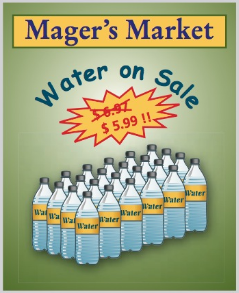Chapter 3, Problem 36AT### Contemporary Mathematics for Busin...

8th Edition
Robert Brechner + 1 other
ISBN: 9781305585447

#### Solutions

Chapter
Section### Contemporary Mathematics for Busin...

8th Edition
Robert Brechner + 1 other
ISBN: 9781305585447
Textbook Problem

# At Mager’s Market, a 24-bottle case of spring water is on sale for $5.99. If the regular price for the case is$6.97,a. How much is saved if a customer buys the case at the sale price?b. What is the sale price per bottle? Round to the nearest cent.c. Which sales strategy earns more revenue for Mager’s Market, selling 400 cases of water per week at the sale price or selling 300 cases per week at the regular price?(a)

To determine

To calculate: The amount a costumer saves if he buys the case at the sale price, if the sale price of the 24-bottle case is $5.99 and the regular prize is$6.97.

Explanation

Given Information:

The sale price and actual price of 24-bottle case of spring water at Mager’s Market is 5.99 and 6.97. Formula used: To subtract two decimal numbers use the steps: Step 1: Align the decimal points vertically. Step 2: Put 0 at the empty places to make the number of digits after and decimal point equal. Step 3: Perform subtraction from right to left and keep decimal point aligned. Calculation: Consider the sales price and the actual prize as5.99 and 6.97 respectively...

(b)

To determine

To calculate: The sale price of each bottle, if the sale price of the 24 bottle case is $5.99 and the regular prize is$6.97.

(c)

To determine

The strategy to that earns more revenue among the strategies as “selling 400 cases of water per week at the sale price or selling 300 cases per week at the regular price” if the sale price of the 24 bottle case is $5.99 and the regular prize is$6.97.

### Still sussing out bartleby?

Check out a sample textbook solution.

See a sample solution

#### The Solution to Your Study Problems

Bartleby provides explanations to thousands of textbook problems written by our experts, many with advanced degrees!

Get Started